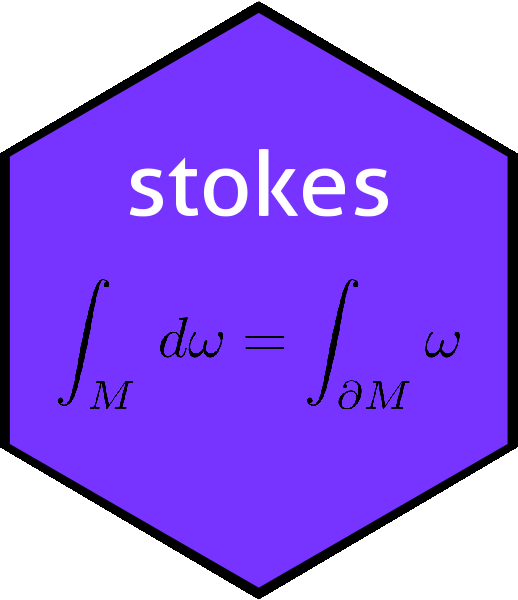# The dovs() function in the stokes package: the dimension of the underlying vector spacedovs
## function (K)
## {
##     max(index(K))
## }
## <bytecode: 0x55abc1bff3f0>
## <environment: namespace:stokes>

Function dovs() returns the dimensionality of the underlying vector space of a $$k$$-form. Recall that a $$k$$-form is an alternating linear map from $$V^k$$ to $$\mathbb{R}$$, where $$V=\mathbb{R}^n$$. Function dovs() returns $$n$$. The function is, as seen above, very simple but its use is not entirely straightforward in the context of stokes idiom.

Consider the following:

set.seed(0)
a <- rform(n=4,k=2)
a
## An alternating linear map from V^2 to R with V=R^4:
##          val
##  2 4  =    9
##  1 4  =    8
##  2 3  =    1
##  1 3  =   -3
##  3 4  =   -2
##  1 2  =    2

Now object a is notionally a map from $$\left(\mathbb{R}^4\right)^2$$ to $$\mathbb{R}$$:

f <- as.function(a)
(M <- matrix(1:8,4,2))
##      [,1] [,2]
## [1,]    1    5
## [2,]    2    6
## [3,]    3    7
## [4,]    4    8
f(M)
##  -148

However, a can equally be considered to be a map from $$\left(\mathbb{R}^5\right)^2$$ to $$\mathbb{R}$$:

f <- as.function(a)
(M <- matrix(1:10,5,2))
##      [,1] [,2]
## [1,]    1    6
## [2,]    2    7
## [3,]    3    8
## [4,]    4    9
## [5,]    5   10
f(M)
##  -185

If we view $$a$$ [or indeed f()] in this way, that is $$a\colon\left(\mathbb{R}^5\right)^2\longrightarrow\mathbb{R}$$, we observe that $$e_5$$, that is $$\left(0,0,0,0,1\right)^T$$, maps to zero:

(M <- cbind(c(0,0,0,0,1),runif(5)))
##      [,1]      [,2]
## [1,]    0 0.3800352
## [2,]    0 0.7774452
## [3,]    0 0.9347052
## [4,]    0 0.2121425
## [5,]    1 0.6516738
f(M)
##  0

(and of course, because a is alternating, we could have put $$e_5$$ in the second column with the same result). The $$k$$form a returns zero because the index matrix of a does not include the number 5.

Most of the time, this does not matter. However, consider this:

dx
## An alternating linear map from V^1 to R with V=R^1:
##        val
##  1  =    1

Now, we know that dx is supposed to be a map from $$\left(\mathbb{R}^3\right)^1$$ to $$\mathbb{R}$$; but:

dovs(dx)
##  1

So according to stokes, $$\operatorname{dx}\colon\left(\mathbb{R}^1\right)^1\longrightarrow\mathbb{R}$$. This does not really matter numerically, until we consider the Hodge star operator. We know that $$\star\operatorname{dx}=\operatorname{dy}\wedge\operatorname{dz}$$, but

hodge(dx)
##  1

R gives, correctly, that the Hodge star of $$\operatorname{dx}$$ is the zero-dimensional volume element (otherwise known as ‘’1’’). To get the right answer, we need to specify dovs explicitly:

hodge(dx,3)
## An alternating linear map from V^2 to R with V=R^3:
##          val
##  2 3  =    1

Actually this looks a lot better with a more appropriate print method:

options(kform_symbolic_print="dx")
hodge(dx,3)
## An alternating linear map from V^2 to R with V=R^3:
##  + dy^dz